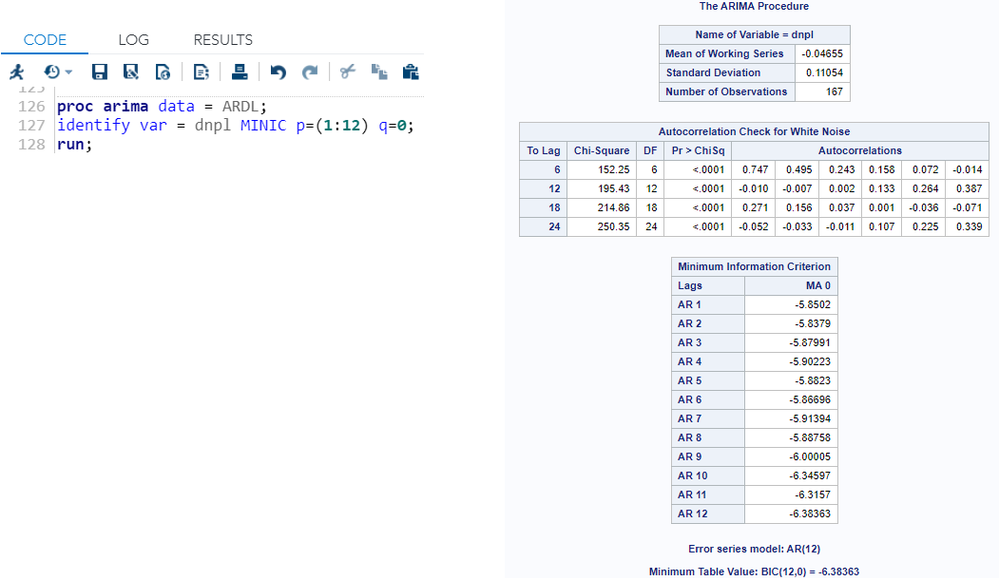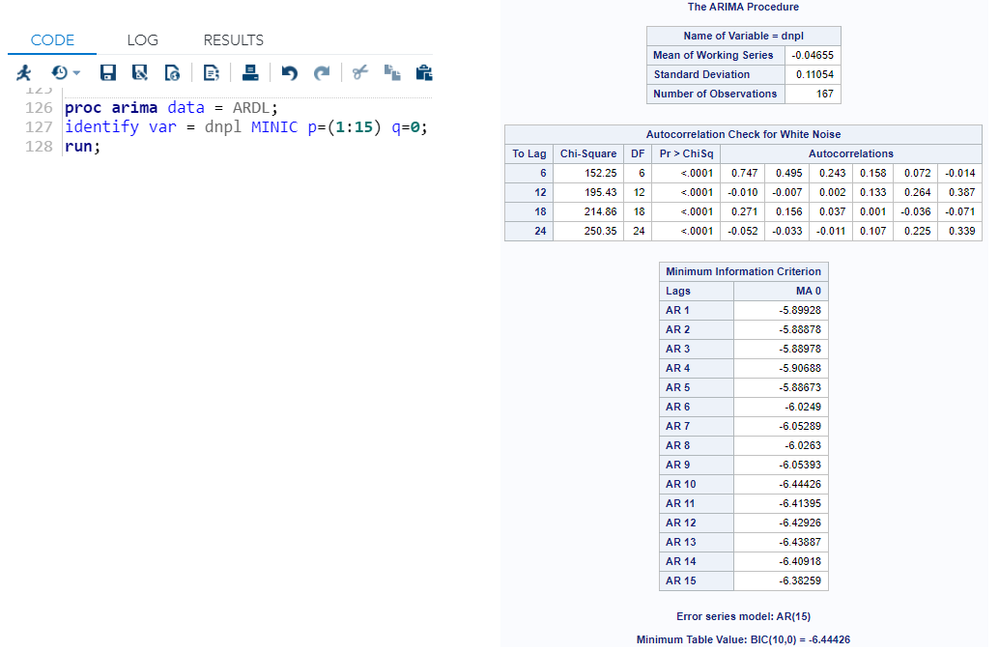## proc ARIMA to identify optimal lag

Hello,

I have 2 questions about proc ARIMA.   I hope somebody can help me out.

(1)  I have tried to find optimal lag length of ARMA process by using  procedure (a) and (b)

Why the minimum information criterion changing when we change p in proc ARIMA?

Procedure (a) :   p = 1, 2, ..., 12     -> This lead to indicate 12 as optimal lag.Procedure (b) :   p = 1, 2, ..., 12  -> This lead to indicate 10 as optimal lag.(2)  How Can I add intercept and  trend in this procedure?

Thank You.

1 ACCEPTED SOLUTION

Accepted Solutions

## Re: proc ARIMA to identify optimal lag

Hi @Golf ,

The difference in the results returned by the MINIC option in your two PROC ARIMA steps is due to a difference in the approximation used for the error series.  In the first PROC ARIMA step, the error series model was an AR(12) model, whereas in the second PROC ARIMA step, the error series model was an AR(15) model.  If you include the PERROR= option with the same specification in both IDENTIFY statements, then the Minimum Information Criterion values will the the same through AR lag 12.  For example:

```proc arima data = ARDL;
identify var = dnpl MINIC p=(1:12) q=0 perror=(10:12);
identify var = dnpl MINIC p=(1:15) q=0 perror=(10:12);
run;```

Regarding your question on how to "add intercept and trend to this procedure", I assume you mean to the underlying procedure used by the MINIC option.  The methodology used by the MINIC option automatically corrects for the mean, however, it assumes the series is stationary (ie. constant mean and variance).  If your series is non-stationary, then you can include a differencing specification in the VAR= option to detrend the series.  The MINIC option operates on the differenced series.  If your series has a deterministic trend component, then you will need to detrend the series prior to running PROC ARIMA with the MINIC option.

I hope this helps!

DW

3 REPLIES 3

## Re: proc ARIMA to identify optimal lag

Hi @Golf ,

The difference in the results returned by the MINIC option in your two PROC ARIMA steps is due to a difference in the approximation used for the error series.  In the first PROC ARIMA step, the error series model was an AR(12) model, whereas in the second PROC ARIMA step, the error series model was an AR(15) model.  If you include the PERROR= option with the same specification in both IDENTIFY statements, then the Minimum Information Criterion values will the the same through AR lag 12.  For example:

```proc arima data = ARDL;
identify var = dnpl MINIC p=(1:12) q=0 perror=(10:12);
identify var = dnpl MINIC p=(1:15) q=0 perror=(10:12);
run;```

Regarding your question on how to "add intercept and trend to this procedure", I assume you mean to the underlying procedure used by the MINIC option.  The methodology used by the MINIC option automatically corrects for the mean, however, it assumes the series is stationary (ie. constant mean and variance).  If your series is non-stationary, then you can include a differencing specification in the VAR= option to detrend the series.  The MINIC option operates on the differenced series.  If your series has a deterministic trend component, then you will need to detrend the series prior to running PROC ARIMA with the MINIC option.

I hope this helps!

DW

## Re: proc ARIMA to identify optimal lag

Thank You so much.

## Re: proc ARIMA to identify optimal lag

You are most welcome, @Golf.  Happy to help!

Discussion stats
• 3 replies
• 919 views
• 2 likes
• 2 in conversation